# Creating Publication-Quality Graphics

## Overview

Teaching: 45 min
Exercises: 20 min
Questions
• How can I create publication-quality graphics in R?

Objectives
• To be able to use ggplot2 to generate publication quality graphics.

• To understand the basic grammar of graphics, including the aesthetics and geometry layers, adding statistics, transforming scales, and coloring or panelling by groups.

Plotting our data is one of the best ways to quickly explore it and the various relationships between variables.

There are three main plotting systems in R, the base plotting system, the lattice package, and the ggplot2 package.

Today we’ll be learning about the ggplot2 package, because it is the most effective for creating publication quality graphics.

ggplot2 is built on the grammar of graphics (where the gg comes from), the idea that any plot can be expressed from the same set of components: a data set, a coordinate system, and a set of geoms–the visual representation of data points.

The key to understanding ggplot2 is thinking about a figure in layers. This idea may be familiar to you if you have used image editing programs like Photoshop, Illustrator, or Inkscape.

Let’s start off with an example. The first thing we need to do is load the `ggplot2` package, just like we did with the previous ones:

``````library("ggplot2")
``````

In order to begin graphing, we use the `ggplot` function. This function lets R know that we’re creating a new plot, and any of the arguments we give the `ggplot` function are the global options for the plot: they apply to all layers on the plot.

By itself, the call to `ggplot` isn’t enough to draw a figure:

``````ggplot(data = gapminder, aes(x = gdpPercap, y = lifeExp))
``````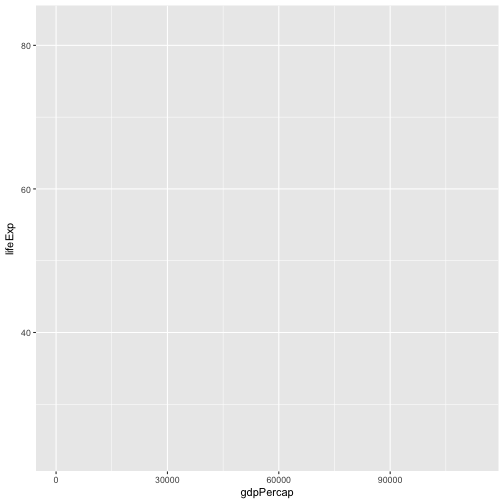We need to tell `ggplot` how we want to visually represent the data, which we do by adding a new geom layer. In our example, we used `geom_point`, which tells `ggplot` we want to visually represent the relationship between x and y as a scatterplot of points:

``````ggplot(data = gapminder, aes(x = gdpPercap, y = lifeExp)) +
geom_point()
``````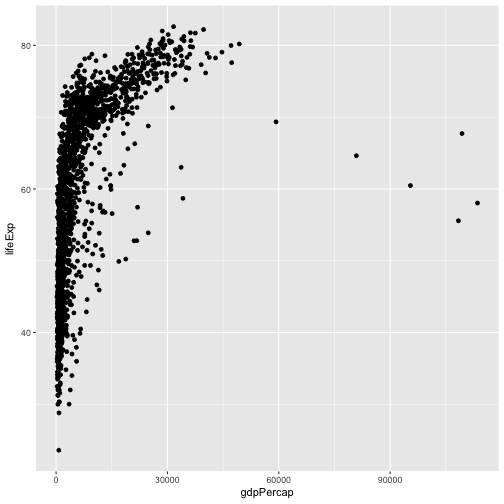We’ve passed in two arguments to `ggplot`. First, we tell `ggplot` what data we want to show on our figure, in this example the gapminder data we read in earlier. For the second argument we passed in the `aes` function, which tells `ggplot` how variables in the data map to aesthetic properties of the figure, in this case the x and y locations. Here we told `ggplot` we want to plot the “gdpPercap” column of the gapminder data frame on the x-axis, and the “lifeExp” column on the y-axis. Notice that we didn’t need to explicitly pass `aes` these columns (e.g. `x = gapminder[, "gdpPercap"]`), this is because `ggplot` is smart enough to know to look in the data for that column!

Other options that can be set with the `aes` function include color, size, transparency and shape. We will talk more about that later.

## Challenge 1

Our example visualizes how the GDP per capita changes in relationship to life expectancy:

``````ggplot(data = gapminder, aes(x = gdpPercap, y = lifeExp)) + geom_point()
``````

Modify this example so that the plot visualizes how life expectancy has changed over time:

Hint: the gapminder dataset has a column called “year”, which should appear on the x-axis.

## Solution to Challenge 1

Modify the example so that the figure visualise how life expectancy has changed over time:

``````ggplot(data = gapminder, aes(x = year, y = lifeExp)) + geom_point()
``````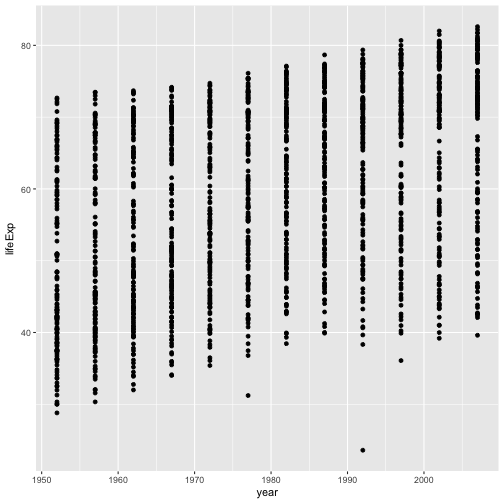## Challenge 2

In the previous examples and challenge we’ve used the `aes` function to tell the scatterplot geom about the x and y locations of each point. Another aesthetic property we can modify is the point color. Modify the code from the previous challenge to color the points by the “continent” column. What trends do you see in the data? Are they what you expected?

## Solution to Challenge 2

In the previous examples and challenge we’ve used the `aes` function to tell the scatterplot geom about the x and y locations of each point. Another aesthetic property we can modify is the point color. Modify the code from the previous challenge to color the points by the “continent” column. What trends do you see in the data? Are they what you expected?

``````ggplot(data = gapminder, aes(x = year, y = lifeExp, color=continent)) +
geom_point()
``````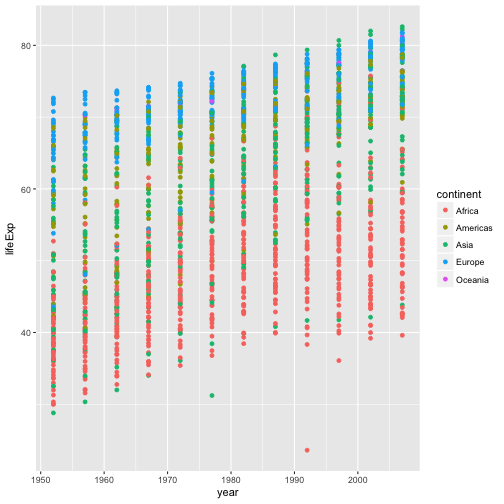## Layers

Using a scatterplot probably isn’t the best for visualizing change over time. Instead, let’s tell `ggplot` to visualize the data as a line plot:

``````ggplot(data = gapminder, aes(x=year, y=lifeExp, by=country, color=continent)) +
geom_line()
``````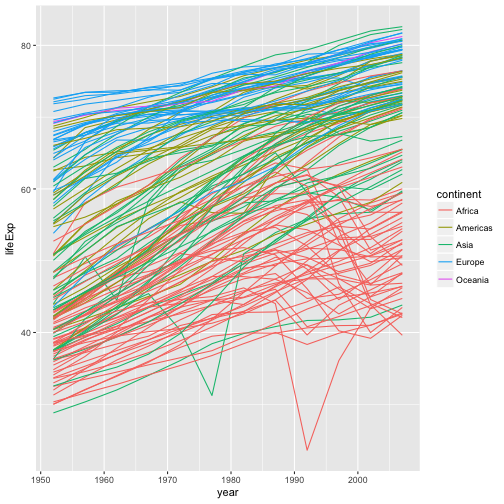Instead of adding a `geom_point` layer, we’ve added a `geom_line` layer. We’ve added the by aesthetic, which tells `ggplot` to draw a line for each country.

But what if we want to visualize both lines and points on the plot? We can simply add another layer to the plot:

``````ggplot(data = gapminder, aes(x=year, y=lifeExp, by=country, color=continent)) +
geom_line() + geom_point()
``````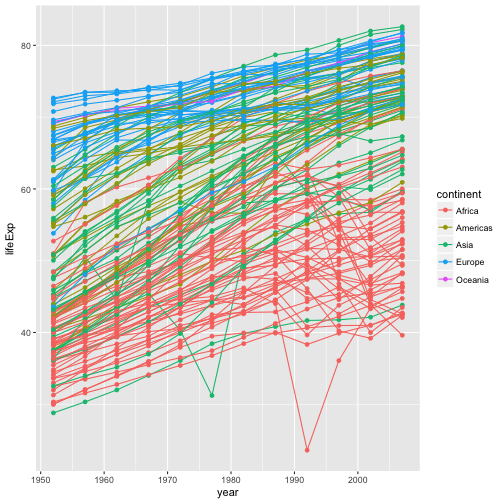It’s important to note that each layer is drawn on top of the previous layer. In this example, the points have been drawn on top of the lines. Here’s a demonstration:

``````ggplot(data = gapminder, aes(x=year, y=lifeExp, by=country)) +
geom_line(aes(color=continent)) + geom_point()
``````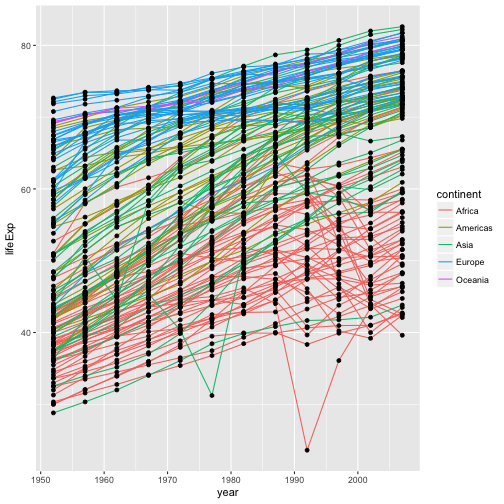In this example, the aesthetic mapping of color has been moved from the global plot options in `ggplot` to the `geom_line` layer so it no longer applies to the points. Now we can clearly see that the points are drawn on top of the lines.

## Tip: Setting an aesthetic to a value instead of a mapping

So far, we’ve seen how to use an aesthetic (such as color) as a mapping to a variable in the data. For example, when we use `geom_line(aes(color=continent))`, ggplot will give a different color to each continent. But what if we want to change the colour of all lines to blue? You may think that `geom_line(aes(color="blue"))` should work, but it doesn’t. Since we don’t want to create a mapping to a specific variable, we simply move the color specification outside of the `aes` function, like this: `geom_line(color="blue")`.

We can further demonstrate this point by switching the order of the point and line layers from the previous example.

``````ggplot(data = gapminder, aes(x=year, y=lifeExp, by=country)) +
geom_point() + geom_line(aes(color=continent))
``````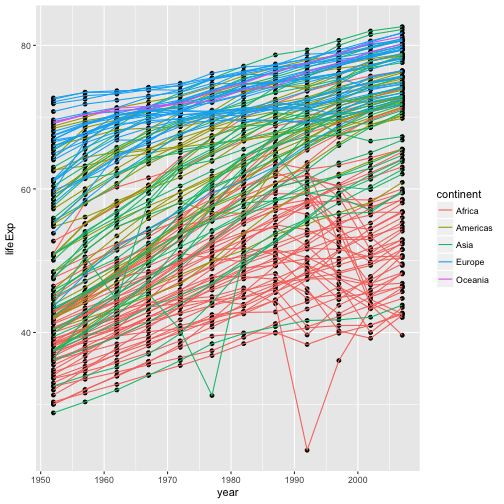The lines now get drawn over the points!

## Transformations and statistics

`ggplot` also makes it easy to overlay statistical models over the data. To demonstrate we’ll go back to our first example:

``````ggplot(data = gapminder, aes(x = gdpPercap, y = lifeExp)) +
geom_point()
``````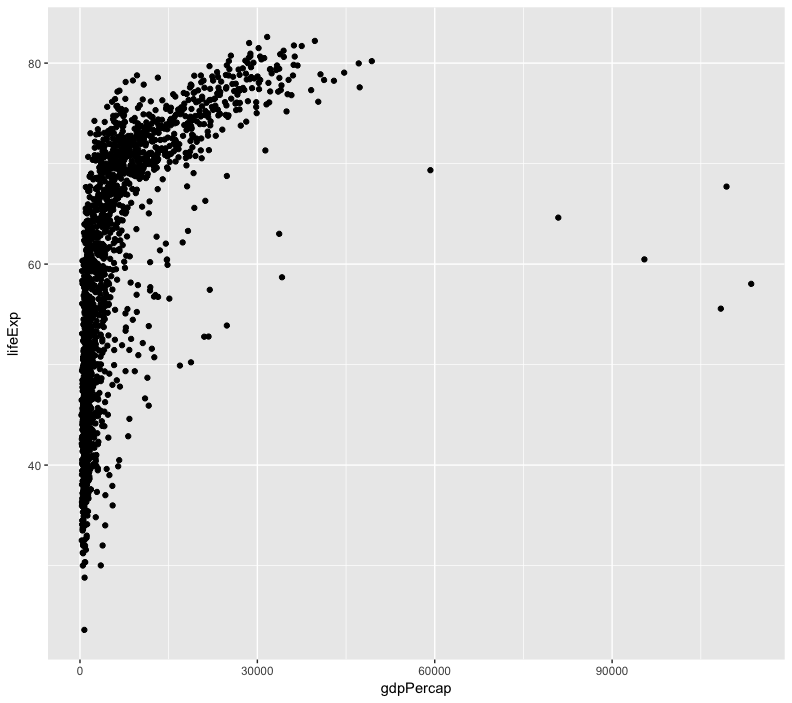Currently it’s hard to see the relationship between the points due to some strong outliers in GDP per capita. We can change the scale of units on the x axis using the scale functions. These control the mapping between the data values and visual values of an aesthetic. We can also modify the transparency of the points, using the alpha function, which is especially helpful when you have a large amount of data which is very clustered.

``````ggplot(data = gapminder, aes(x = gdpPercap, y = lifeExp)) +
geom_point(alpha = 0.5) + scale_x_log10()
``````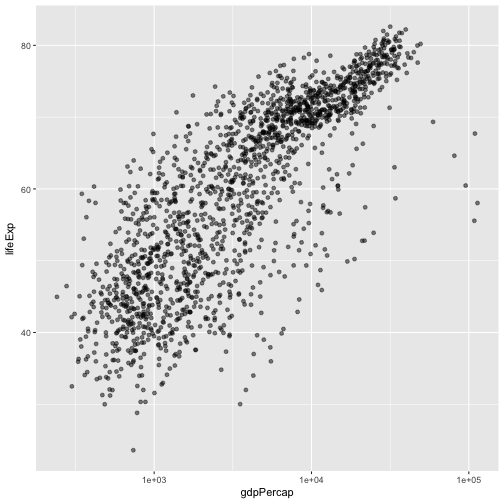The `log10` function applied a transformation to the values of the gdpPercap column before rendering them on the plot, so that each multiple of 10 now only corresponds to an increase in 1 on the transformed scale, e.g. a GDP per capita of 1,000 is now 3 on the y axis, a value of 10,000 corresponds to 4 on the y axis and so on. This makes it easier to visualize the spread of data on the x-axis.

## Tip Reminder: Setting an aesthetic to a value instead of a mapping

Notice that we used `geom_point(alpha = 0.5)`. As the previous tip mentioned, using a setting outside of the `aes` function will cause this value to be used for all points, which is what we want in this case. But just like any other aesthetic setting, alpha can also be mapped to a variable in the data. For example, we can give a different transparency to each continent with `geom_point(aes(alpha = continent))`.

We can fit a simple relationship to the data by adding another layer, `geom_smooth`:

``````ggplot(data = gapminder, aes(x = gdpPercap, y = lifeExp)) +
geom_point() + scale_x_log10() + geom_smooth(method="lm")
``````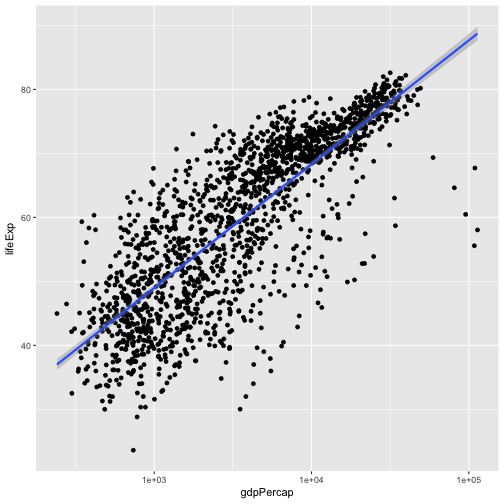We can make the line thicker by setting the size aesthetic in the `geom_smooth` layer:

``````ggplot(data = gapminder, aes(x = gdpPercap, y = lifeExp)) +
geom_point() + scale_x_log10() + geom_smooth(method="lm", size=1.5)
``````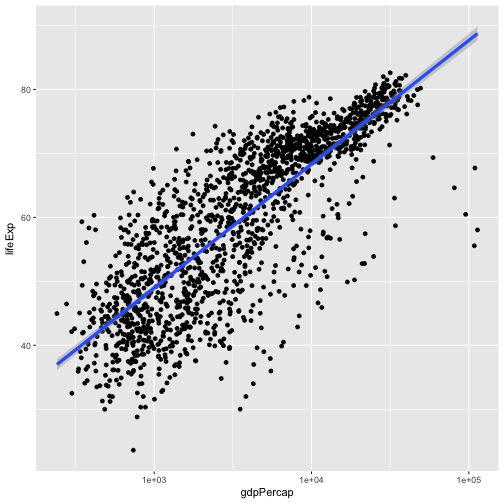There are two ways an aesthetic can be specified. Here we set the size aesthetic by passing it as an argument to `geom_smooth`. Previously in the lesson we’ve used the `aes` function to define a mapping between data variables and their visual representation.

## Challenge 3

Modify the color and size of the points on the point layer in the previous example.

Hint: do not use the `aes` function, change this by adding arguments to the correct function.

## Solution to Challenge 3

Since we want all the points to be the same and are not making this aesthetic specific to the data, we add this to `geom_point` to make the change effect all points but not the line.

``````ggplot(data = gapminder, aes(x = gdpPercap, y = lifeExp)) +
geom_point(size=3, color="orange") + scale_x_log10() +
geom_smooth(method="lm", size=1.5)
``````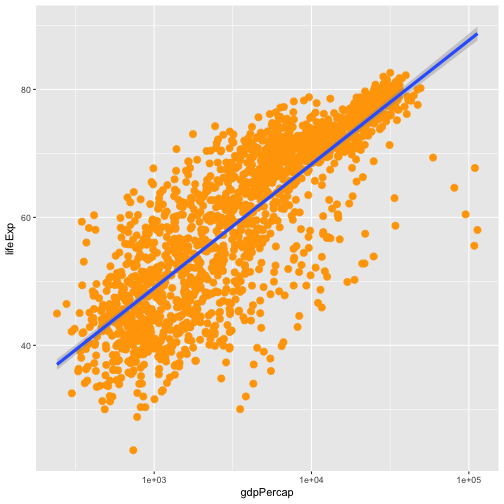## Challenge 4

Modify your solution to Challenge 3 so that the points are now a different shape and are colored by continent with new trendlines.

Hint: The color argument can be used inside the aesthetic. To change the shape of a point, use the `pch` argument. Setting `pch` to different numeric values from `1:25` yields different shapes as indicated in the chart below.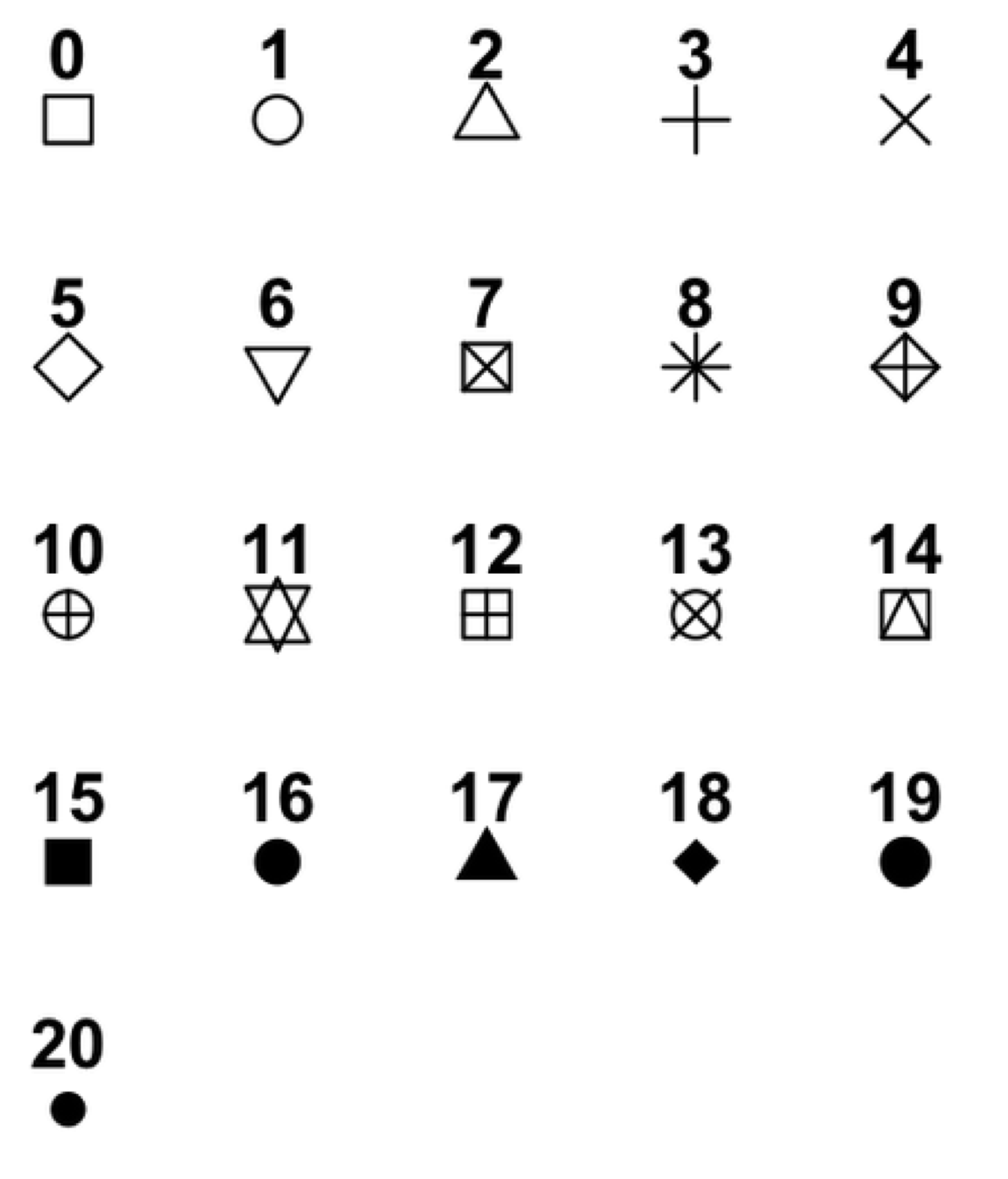## Solution to Challenge 4

Since we want the color to be dependent on the continent, we place that argument inside the `aes`. To change the shape of the point, we place the `pch` argument inside `geom_point`.

``````ggplot(data = gapminder, aes(x = gdpPercap, y = lifeExp, color = continent)) +
geom_point(size=3, pch=17) + scale_x_log10() +
geom_smooth(method="lm", size=1.5)
``````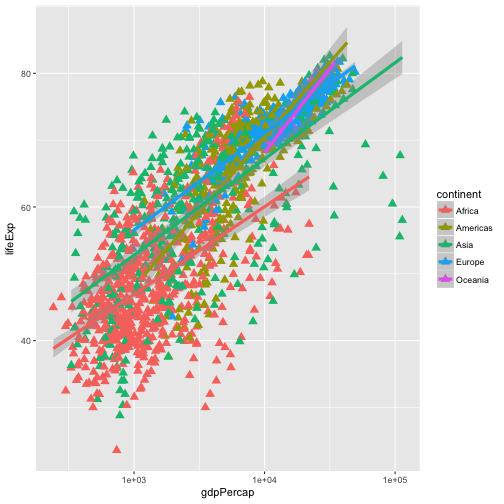## Multi-panel figures

Earlier we visualized the change in life expectancy over time across all countries in one plot. Alternatively, we can split this out over multiple panels by adding a layer of facet panels. Focusing only on those countries with names that start with the letter “A” or “Z”.

We start by subsetting the data. We use the `substr` function to pull out a part of a character string; in this case, the letters that occur in positions `start` through `stop`, inclusive, of the `gapminder\$country` vector. As we saw previously, the `%in%` operator allows us to make multiple comparisons rather than write out long subsetting conditions (in this case, `starts.with %in% c("A", "Z")` is equivalent to `starts.with == "A" | starts.with == "Z"`)

``````starts.with <- substr(gapminder\$country, start = 1, stop = 1)
az.countries <- gapminder[starts.with %in% c("A", "Z"), ]
ggplot(data = az.countries, aes(x = year, y = lifeExp, color=continent)) +
geom_line() + facet_wrap( ~ country)
``````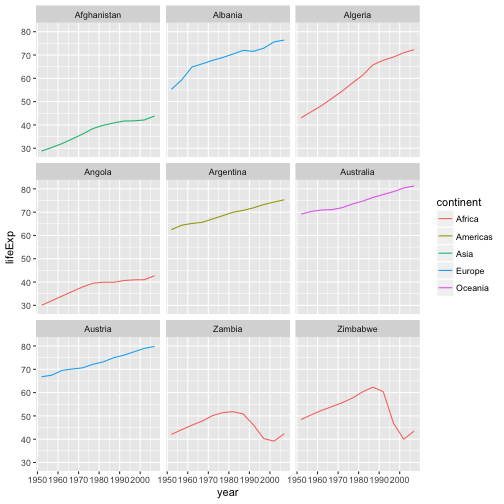The `facet_wrap` layer takes a “formula” as its argument, denoted by the tilde (~). This tells R to draw a panel for each unique value in the country column of the gapminder dataset.

## Modifying text

To clean this figure up for a publication we need to change some of the text elements.

First, let’s rename our `x` and `y` axes to neater and more informative labels. We can do that using the `xlab` and `ylab` functions:

``````ggplot(data = az.countries, aes(x = year, y = lifeExp, color=continent)) +
geom_line() + facet_wrap( ~ country) +
xlab("Year") + ylab("Life Expectancy")
``````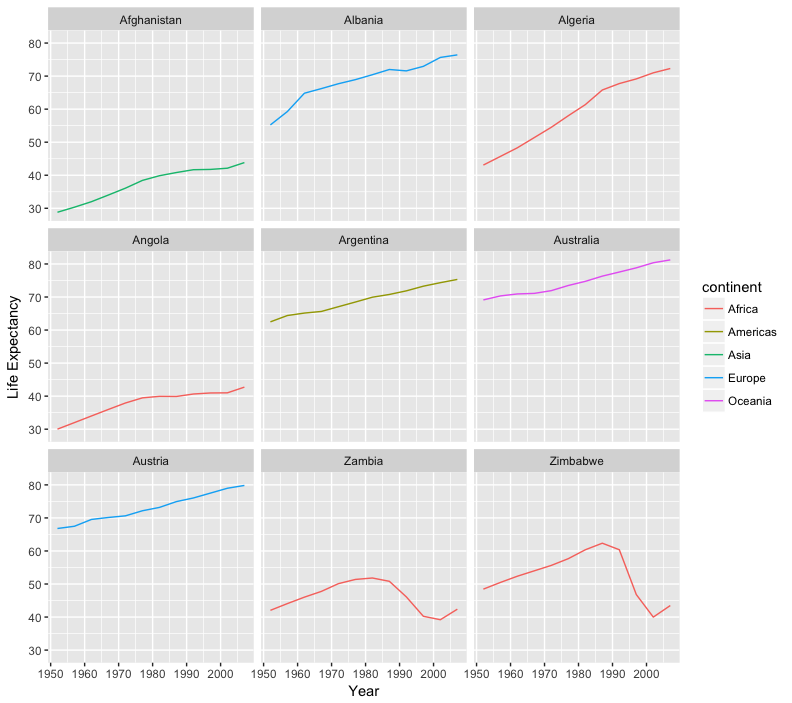Let’s give our figure a title with the `ggtitle` function. And while we’re at it, let’s capitalize the label of our legend. This can be done using the scales layer.

``````ggplot(data = az.countries, aes(x = year, y = lifeExp, color=continent)) +
geom_line() + facet_wrap( ~ country) +
xlab("Year") + ylab("Life Expectancy") +
ggtitle("Figure 1") + scale_colour_discrete(name="Continent")
``````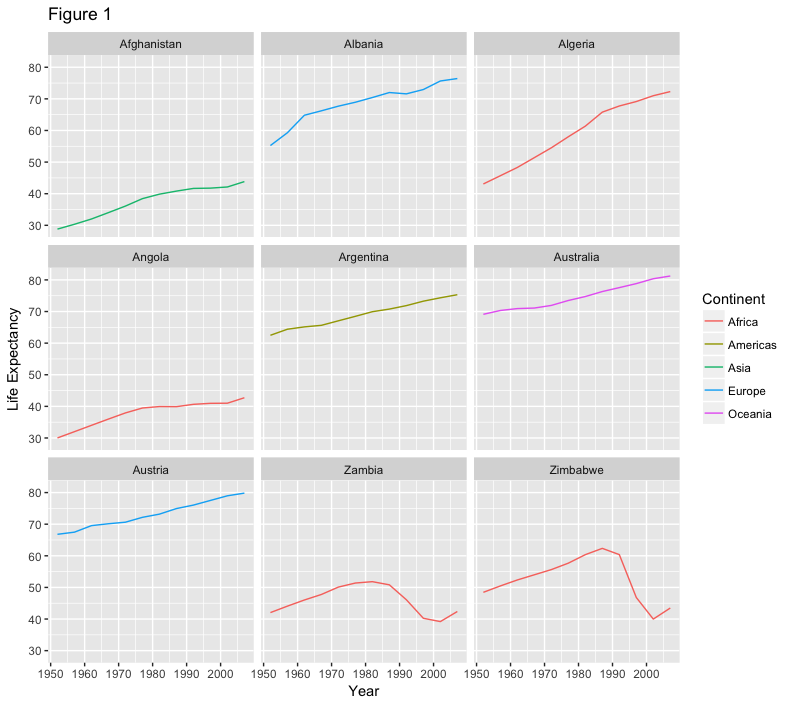Lastly, let’s remove the x-axis labels so the plot is less cluttered. To do this, we use the theme layer which controls the axis text and overall text size.

``````ggplot(data = az.countries, aes(x = year, y = lifeExp, color=continent)) +
geom_line() + facet_wrap( ~ country) +
xlab("Year") + ylab("Life Expectancy") +
ggtitle("Figure 1") + scale_colour_discrete(name="Continent") +
theme(axis.text.x=element_blank(), axis.ticks.x=element_blank())
``````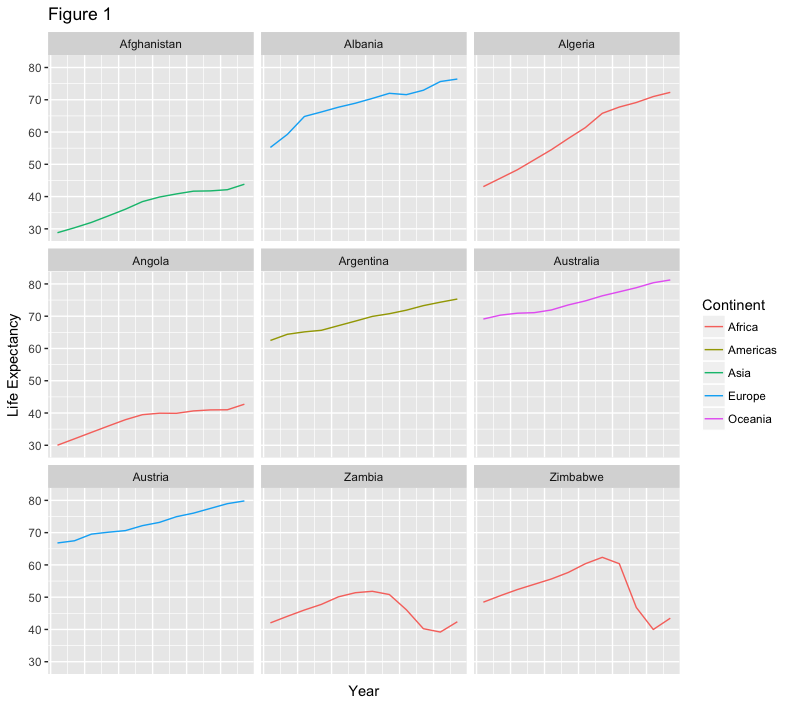This is a taste of what you can do with `ggplot2`. RStudio provides a really useful cheat sheet of the different layers available, and more extensive documentation is available on the ggplot2 website. Finally, if you have no idea how to change something, a quick Google search will usually send you to a relevant question and answer on Stack Overflow with reusable code to modify!

## Challenge 5

Create a density plot of GDP per capita, filled by continent.

• Transform the x axis to better visualise the data spread.
• Add a facet layer to panel the density plots by year.

## Solution to Challenge 5

Create a density plot of GDP per capita, filled by continent.

``````ggplot(data = gapminder, aes(x = gdpPercap, fill=continent)) +
geom_density(alpha=0.6)
``````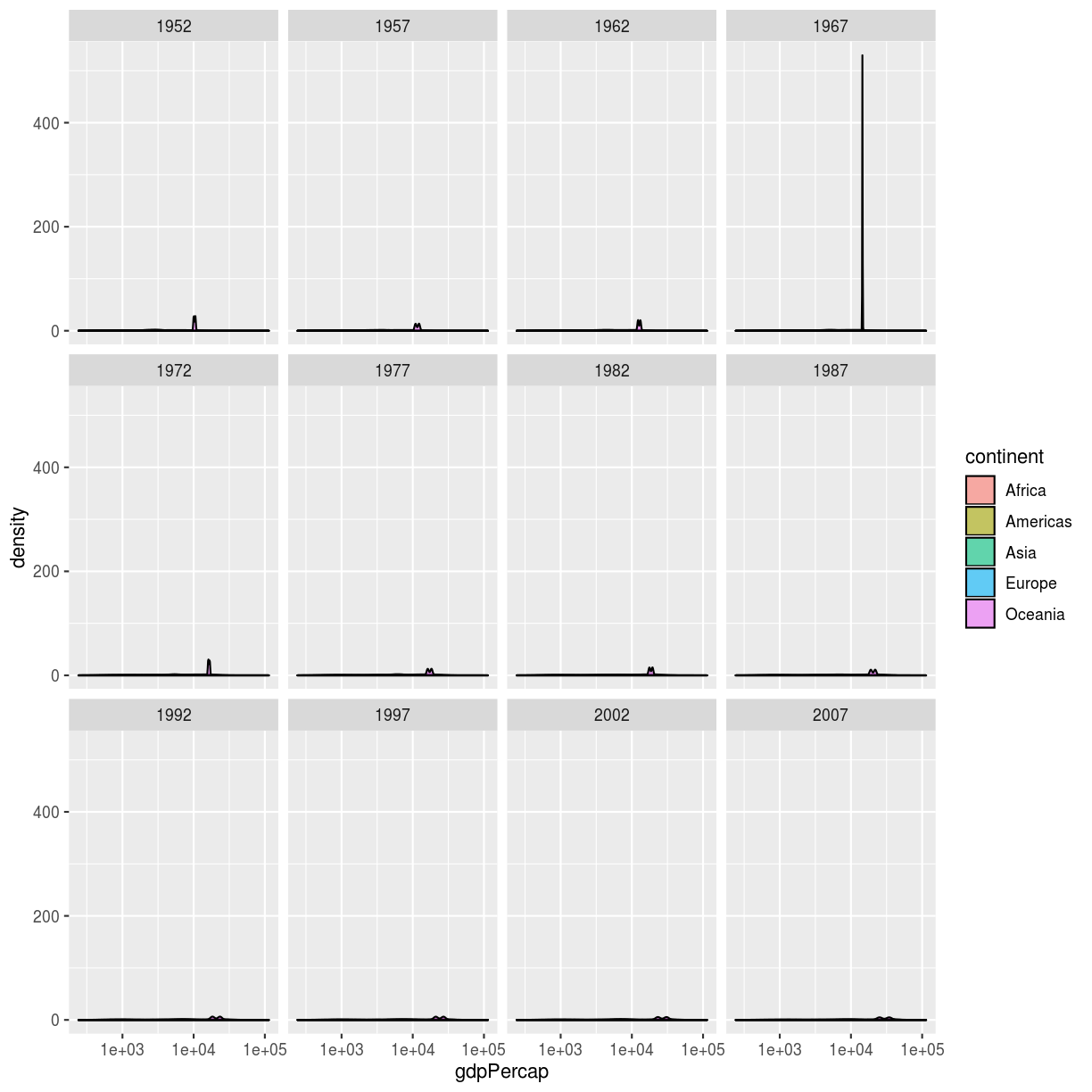• Transform the x axis to better visualise the data spread.
• Add a facet layer to panel the density plots by year.
``````ggplot(data = gapminder, aes(x = gdpPercap, fill=continent)) +
geom_density(alpha=0.6) + facet_wrap( ~ year) + scale_x_log10()
``````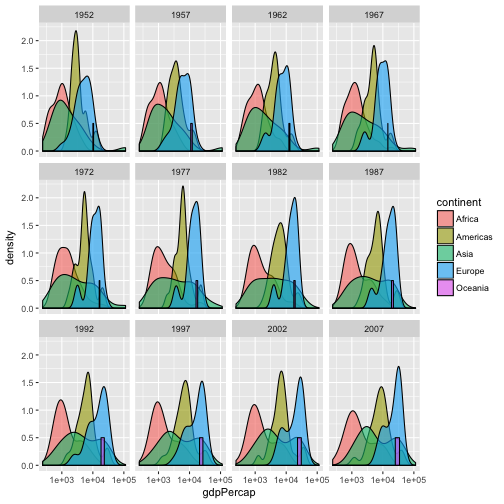## Key Points

• Use `ggplot2` to create plots.

• Think about graphics in layers: aesthetics, geometry, statistics, scale transformation, and grouping.